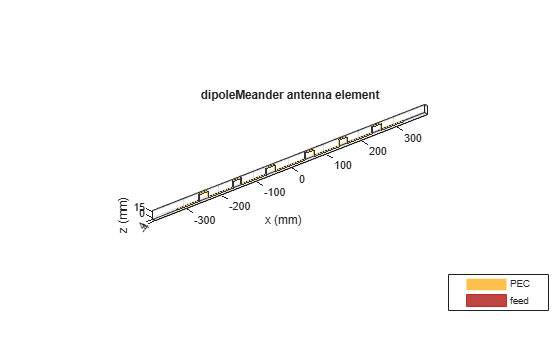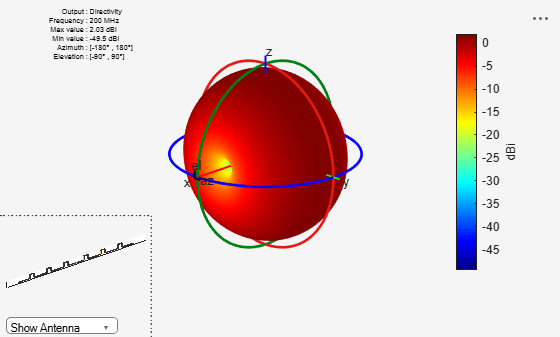# dipoleMeander

Create meander dipole antenna

## Description

The `dipoleMeander` class creates a meander dipole antenna with four dipoles. The antenna is center fed and it is symmetric about its origin. The first resonance of meander dipole antenna is at 200 MHz.

The width of the dipole is related to the diameter of an equivalent cylindrical dipole by the equation

`$w=2d=4r$`

, where:

• d is the diameter of equivalent cylindrical dipole.

• r is the radius of equivalent cylindrical dipole.

For a given cylinder radius, use the `cylinder2strip` utility function to calculate the equivalent width. The default strip dipole is center-fed. The feed point coincides with the origin. The origin is located on the xy- plane.## Creation

### Syntax

``dm = dipoleMeander``
``dm = dipoleMeander(Name,Value)``

### Description

example

``` `dm = dipoleMeander` creates a meander dipole antenna with four dipoles.```
``` `dm = dipoleMeander(Name,Value)` creates a meander dipole antenna with four dipoles, with additional properties specified by one or more name-value pair arguments. `Name` is the property name and `Value` is the corresponding value. You can specify several name-value pair arguments in any order as `Name1`, `Value1`, `...`, `NameN`, `ValueN`. Properties not specified retain their default values.```

## Properties

expand all

Dipole width, specified as a scalar in meters.

Example: `'Width',0.05`

Data Types: `double`

Length of individual dipole arms, specified as a vector in meters. The total number of dipole arms generated is :

`$2\ast N-1$`

where N is the number of specified arm lengths.

Example: ```'ArmLength',[0.6000 0.5000 1 0.4000]```

Data Types: `double`

Notch length along the length of the antenna, specified as a scalar in meters.

For example, in a dipole meander antenna with seven stacked arms there are six notches.

Example: `'NotchLength',1`

Data Types: `double`

Notch width perpendicular to the length of the antenna, specified as a scalar in meters.

Example: `'NotchWidth',1`

Data Types: `double`

Type of the metal used as a conductor, specified as a metal material object. You can choose any metal from the `MetalCatalog` or specify a metal of your choice. For more information, see `metal`. For more information on metal conductor meshing, see Meshing.

Example: ```m = metal('Copper'); 'Conductor',m```

Example: ```m = metal('Copper'); ant.Conductor = m```

Lumped elements added to the antenna feed, specified as a lumped element object. For more information, see `lumpedElement`.

Example: `'Load',lumpedelement`. `lumpedelement` is the object for the load created using `lumpedElement`.

Example: ```dm.Load = lumpedElement('Impedance',75)```

Tilt angle of the antenna, specified as a scalar or vector with each element unit in degrees. For more information, see Rotate Antennas and Arrays.

Example: `'Tilt',90`

Example: `ant.Tilt = 90`

Example: `'Tilt',[90 90]`,`'TiltAxis',[0 1 0;0 1 1]` tilts the antenna at 90 degrees about the two axes defined by the vectors.

Note

The `wireStack` antenna object only accepts the dot method to change its properties.

Data Types: `double`

Tilt axis of the antenna, specified as:

• Three-element vector of Cartesian coordinates in meters. In this case, each coordinate in the vector starts at the origin and lies along the specified points on the X-, Y-, and Z-axes.

• Two points in space, each specified as three-element vectors of Cartesian coordinates. In this case, the antenna rotates around the line joining the two points in space.

• A string input describing simple rotations around one of the principal axes, 'X', 'Y', or 'Z'.

Example: `'TiltAxis',[0 1 0]`

Example: `'TiltAxis',[0 0 0;0 1 0]`

Example: `ant.TiltAxis = 'Z'`

Note

The `wireStack` antenna object only accepts the dot method to change its properties.

Data Types: `double`

## Object Functions

 `show` Display antenna or array structure; display shape as filled patch `info` Display information about antenna or array `axialRatio` Axial ratio of antenna `beamwidth` Beamwidth of antenna `charge` Charge distribution on metal or dielectric antenna or array surface `current` Current distribution on metal or dielectric antenna or array surface `design` Design prototype antenna or arrays for resonance around specified frequency `efficiency` Radiation efficiency of antenna `EHfields` Electric and magnetic fields of antennas; Embedded electric and magnetic fields of antenna element in arrays `impedance` Input impedance of antenna; scan impedance of array `mesh` Mesh properties of metal or dielectric antenna or array structure `meshconfig` Change mesh mode of antenna structure `optimize` Optimize antenna or array using SADEA optimizer `pattern` Radiation pattern and phase of antenna or array; Embedded pattern of antenna element in array `patternAzimuth` Azimuth pattern of antenna or array `patternElevation` Elevation pattern of antenna or array `rcs` Calculate and plot radar cross section (RCS) of platform, antenna, or array `returnLoss` Return loss of antenna; scan return loss of array `sparameters` Calculate S-parameter for antenna and antenna array objects `vswr` Voltage standing wave ratio of antenna

## Examples

collapse all

Create and view the default meander dipole antenna.

`dm = dipoleMeander`
```dm = dipoleMeander with properties: Width: 0.0040 ArmLength: [0.0880 0.0710 0.0730 0.0650] NotchLength: 0.0238 NotchWidth: 0.0170 Conductor: [1x1 metal] Tilt: 0 TiltAxis: [1 0 0] Load: [1x1 lumpedElement] ```
`show(dm)`Plot the radiation pattern of meander dipole antenna at a 200 MHz frequency.

```dm = dipoleMeander; pattern(dm,200e6)```Balanis, C.A. Antenna Theory: Analysis and Design. 3rd Ed. New York: Wiley, 2005.